## Linear Regression - Introduction¶Linear regression relates a continuous response (dependent) variable to one or more predictors (features, independent variables), using the assumption that the relationship is linear in nature:

• The relationship between each feature and the response is a straight line when we keep other features constant.
• The slope of this line does not depend on the values of the other variables.
• The effects of each variable on the response are additive (but we can include new variables that represent the interaction of two variables).

The model assumes that the response variable can be explained or predicted by a linear combination of the features, except for random deviations from this linear relationship.

## Imports & Settings¶

In :
%matplotlib inline
from pathlib import Path
import matplotlib.pyplot as plt
import seaborn as sns
import pandas as pd
import numpy as np
from mpl_toolkits.mplot3d import Axes3D
from matplotlib import cm
import statsmodels.stats.api as sms
import statsmodels.api as sm
import statsmodels.tsa.api as smt
from sklearn.linear_model import SGDRegressor
from sklearn.preprocessing import StandardScaler
#from statsmodels.tsa.stattools import acf, q_stat, adfuller
from scipy.stats import probplot, moment
import warnings

In :
plt.style.use('ggplot')
pd.options.display.float_format = '{:,.2f}'.format
warnings.filterwarnings('ignore')

In :
def plotting_3_chart(df, feature):
## Importing seaborn, matplotlab and scipy modules.
import matplotlib.gridspec as gridspec
from scipy import stats

## Creating a customized chart. and giving in figsize and everything.
fig = plt.figure(constrained_layout=True, figsize=(8,5))
## creating a grid of 3 cols and 3 rows.
grid = gridspec.GridSpec(ncols=3, nrows=3, figure=fig)

## Customizing the histogram grid.
## Set the title.
ax1.set_title(f'{feature} Histogram')
## plot the histogram.
sns.distplot(df.loc[:,feature], norm_hist=True, ax = ax1, bins=20, fit=stats.norm)
ax1.legend(('normal', f'{feature}'))

# customizing the QQ_plot.
## Set the title.
ax2.set_title('QQ_plot')
## Plotting the QQ_Plot.
stats.probplot(df.loc[:,feature], plot = ax2)

## Customizing the Box Plot.
## Set title.
ax3.set_title('Box Plot')
## Plotting the box plot.
sns.boxplot(df.loc[:,feature], orient='v', ax = ax3 );

In :
def tsplot(y, lags=None, title='', figsize=(12, 6)):

# Examine the patterns of ACF and PACF, along with the time series plot and histogram.
# Original source: https://tomaugspurger.github.io/modern-7-timeseries.html

fig = plt.figure(figsize=figsize)
layout = (2, 2)
ts_ax   = plt.subplot2grid(layout, (0, 0))
hist_ax = plt.subplot2grid(layout, (0, 1))
acf_ax  = plt.subplot2grid(layout, (1, 0))
pacf_ax = plt.subplot2grid(layout, (1, 1))

y.plot(ax=ts_ax)
ts_ax.set_title(title)
y.plot(ax=hist_ax, kind='hist', bins=30, alpha=.5)
hist_ax.set_title('Histogram')
smt.graphics.plot_acf(y, lags=lags, ax=acf_ax)
smt.graphics.plot_pacf(y, lags=lags, ax=pacf_ax)
[ax.set_xlim(0) for ax in [acf_ax, pacf_ax]]
sns.despine()
plt.tight_layout()

return ts_ax, acf_ax, pacf_ax


### Simple Regression¶

#### Generate random data¶

In :
x = np.linspace(-5, 50, 100)
y = 50 + 2 * x  + np.random.normal(0, 20, size=len(x))
data = pd.DataFrame({'X': x, 'Y': y})
ax = data.plot.scatter(x='X', y='Y');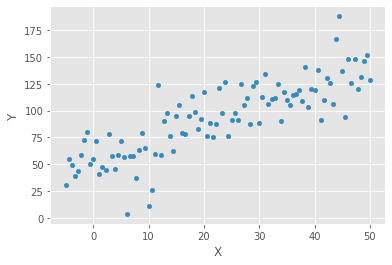Our linear model with a single independent variable on the left-hand side assumes the following form:

$$y = \beta_0 + \beta_1 X_1 + \epsilon$$

$\epsilon$ accounts for the deviations or errors that we will encounter when our data do not actually fit a straight line. When $\epsilon$ materializes, that is when we run the model of this type on actual data, the errors are called residuals.

#### Estimate a regression with statsmodels¶

The upper part of the summary displays the dataset characteristics, namely the estimation method, the number of observations and parameters, and indicates that standard error estimates do not account for heteroskedasticity.

The middle panel shows the coefficient values that closely reflect the artificial data generating process. We can confirm that the estimates displayed in the middle of the summary result can be obtained using the OLS formula:

In :
X = sm.add_constant(data['X'])
model = sm.OLS(data['Y'], X).fit()
print(model.summary())

                            OLS Regression Results
==============================================================================
Dep. Variable:                      Y   R-squared:                       0.691
Method:                 Least Squares   F-statistic:                     219.0
Date:                Tue, 03 Mar 2020   Prob (F-statistic):           1.01e-26
Time:                        18:00:00   Log-Likelihood:                -437.91
No. Observations:                 100   AIC:                             879.8
Df Residuals:                      98   BIC:                             885.0
Df Model:                           1
Covariance Type:            nonrobust
==============================================================================
coef    std err          t      P>|t|      [0.025      0.975]
------------------------------------------------------------------------------
const         52.2605      3.359     15.557      0.000      45.594      58.927
X              1.7991      0.122     14.798      0.000       1.558       2.040
==============================================================================
Omnibus:                        5.826   Durbin-Watson:                   1.838
Prob(Omnibus):                  0.054   Jarque-Bera (JB):                8.048
Skew:                          -0.191   Prob(JB):                       0.0179
Kurtosis:                       4.336   Cond. No.                         47.6
==============================================================================

Warnings:
 Standard Errors assume that the covariance matrix of the errors is correctly specified.


Heteroskedasticity occurs when the residual variance is not constant but differs across observations. If the residual variance is positively correlated with an input variable, that is, when errors are larger for input values that are far from their mean, then OLS standard error estimates will be too low, and, consequently, the t -statistic will be inflated leading to false discoveries of relationships where none actually exist.

Diagnostics starts with a visual inspection of the residuals. Systematic patterns in the (supposedly random) residuals suggest statistical tests of the null hypothesis that errors are homoscedastic against various alternatives. These tests include the Breusch—Pagan and White tests.

There are several ways to correct OLS estimates for heteroskedasticity:

• Robust standard errors (sometimes called white standard errors) take heteroskedasticity into account when computing the error variance using a so-called sandwich estimator.

• Clustered standard errors assume that there are distinct groups in your data that are homoskedastic but the error variance differs between groups. These groups could be different asset classes or equities from different industries.

Several alternatives to OLS estimate the error covariance matrix using different assumptions when there is heteroskedasticity. The following are available in statsmodels:

• Weighted least squares (WLS): For heteroskedastic errors where the covariance matrix has only diagonal entries as for OLS, but now the entries are allowed to vary

• Feasible generalized least squares (GLSAR), for autocorrelated errors that follow an autoregressive AR (p) process (see the chapter on linear time series models)

• Generalized least squares (GLS) for arbitrary covariance matrix structure; yields efficient and unbiased estimates in the presence of heteroskedasticity or serial correlation

#### Verify calculation¶

In :
beta = np.linalg.inv(X.T.dot(X)).dot(X.T.dot(y))
pd.Series(beta, index=X.columns)

Out:
const   52.26
X        1.80
dtype: float64

#### Display model & residuals¶

In :
data['y-hat'] = model.predict()
data['residuals'] = model.resid
ax = data.plot.scatter(x='X', y='Y', c='darkgrey')
data.plot.line(x='X', y='y-hat', ax=ax);
for _, row in data.iterrows():
plt.plot((row.X, row.X), (row.Y, row['y-hat']), 'k-')

res = pd.DataFrame(model.resid, columns=['resid'], index=data.index)
plotting_3_chart(res, 'resid')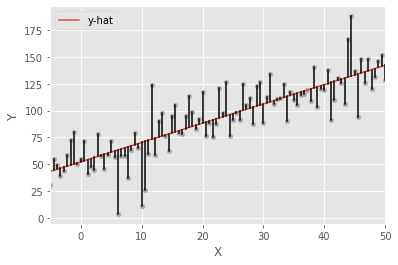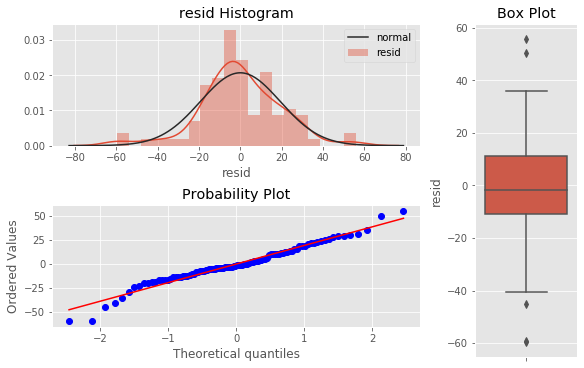In :
tsplot(res, lags=50);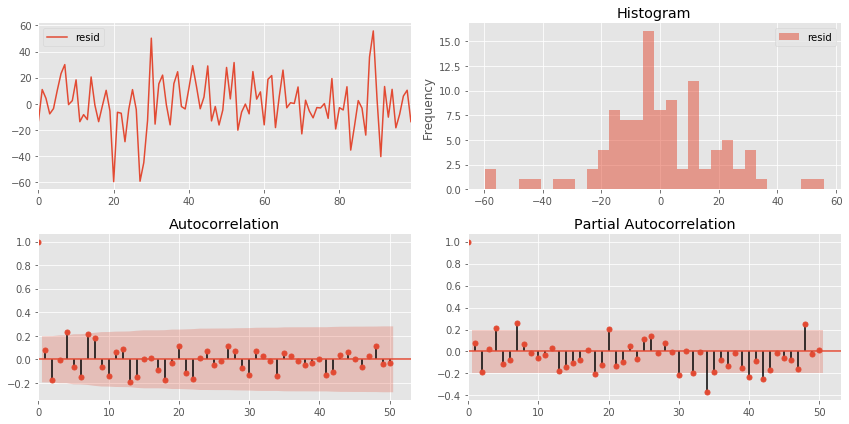### Multiple Regression¶

For two independent variables, the model changes as follows:

$$y = \beta_0 + \beta_1 X_1 + \beta_2 X_2 + \epsilon$$

#### Generate new random data¶

In :
## Create data
size = 25
X_1, X_2 = np.meshgrid(np.linspace(-50, 50, size), np.linspace(-50, 50, size), indexing='ij')
data = pd.DataFrame({'X_1': X_1.ravel(), 'X_2': X_2.ravel()})
data['Y'] = 50 + data.X_1 + 3 * data.X_2 + np.random.normal(0, 50, size=size**2)

## Plot
three_dee = plt.figure(figsize=(15, 5)).gca(projection='3d')
three_dee.scatter(data.X_1, data.X_2, data.Y, c='g');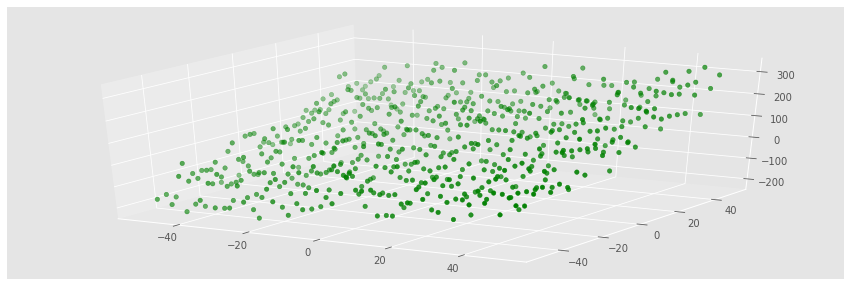In :
X = data[['X_1', 'X_2']]
y = data['Y']


#### Estimate multiple regression model with statsmodels¶

The upper right part of the panel displays the goodness-of-fit measures just discussed, alongside the F-test that rejects the null hypothesis that all coefficients are zero and irrelevant. Similarly, the t-statistics indicate that intercept and both slope coefficients are, highly significant.

The bottom part of the summary contains the residual diagnostics. The left panel displays skew and kurtosis that are used to test the normality hypothesis. Both the Omnibus and the Jarque—Bera test fails to reject the null hypothesis that the residuals are normally distributed. The Durbin—Watson statistic tests for serial correlation in the residuals and has a value near 2 which, given 2 parameters and 625 observations, fails to reject the hypothesis of no serial correlation.

Lastly, the condition number provides evidence about multicollinearity: it is the ratio of the square roots of the largest and the smallest eigenvalue of the design matrix that contains the input data. A value above 30 suggests that the regression may have significant multicollinearity.

In :
X_ols = sm.add_constant(X)
model = sm.OLS(y, X_ols).fit()
print(model.summary())

                            OLS Regression Results
==============================================================================
Dep. Variable:                      Y   R-squared:                       0.804
Method:                 Least Squares   F-statistic:                     1278.
Date:                Tue, 03 Mar 2020   Prob (F-statistic):          5.00e-221
Time:                        18:00:07   Log-Likelihood:                -3303.4
No. Observations:                 625   AIC:                             6613.
Df Residuals:                     622   BIC:                             6626.
Df Model:                           2
Covariance Type:            nonrobust
==============================================================================
coef    std err          t      P>|t|      [0.025      0.975]
------------------------------------------------------------------------------
const         50.5597      1.916     26.393      0.000      46.798      54.322
X_1            1.0409      0.064     16.327      0.000       0.916       1.166
X_2            3.0506      0.064     47.848      0.000       2.925       3.176
==============================================================================
Omnibus:                        0.016   Durbin-Watson:                   1.965
Prob(Omnibus):                  0.992   Jarque-Bera (JB):                0.063
Skew:                          -0.006   Prob(JB):                        0.969
Kurtosis:                       2.952   Cond. No.                         30.0
==============================================================================

Warnings:
 Standard Errors assume that the covariance matrix of the errors is correctly specified.


#### Verify computation¶

In :
beta = np.linalg.inv(X_ols.T.dot(X_ols)).dot(X_ols.T.dot(y))
pd.Series(beta, index=X_ols.columns)

Out:
const   50.56
X_1      1.04
X_2      3.05
dtype: float64

#### Save output as image¶

In :
plt.rc('figure', figsize=(12, 7))
plt.text(0.01, 0.05, str(model.summary()), {'fontsize': 14}, fontproperties = 'monospace')
plt.axis('off')
plt.tight_layout()
# plt.savefig('multiple_regression_summary.png', bbox_inches='tight', dpi=300);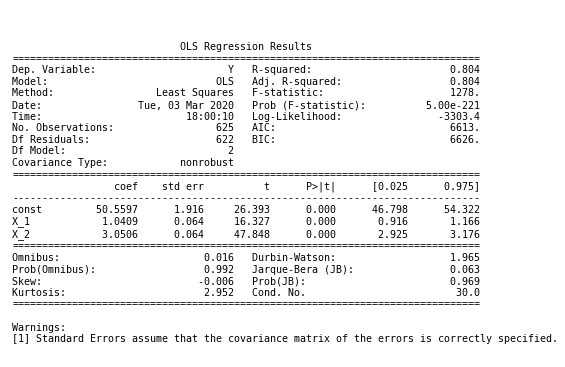#### Display model & residuals¶

In :
res = pd.DataFrame(model.resid, columns=['resid'], index=data.index)
plotting_3_chart(res, 'resid')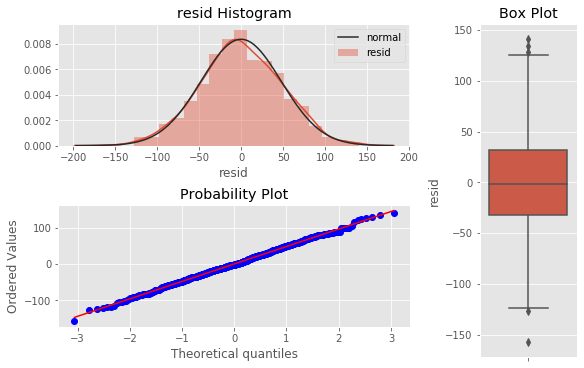In :
tsplot(res, lags=50);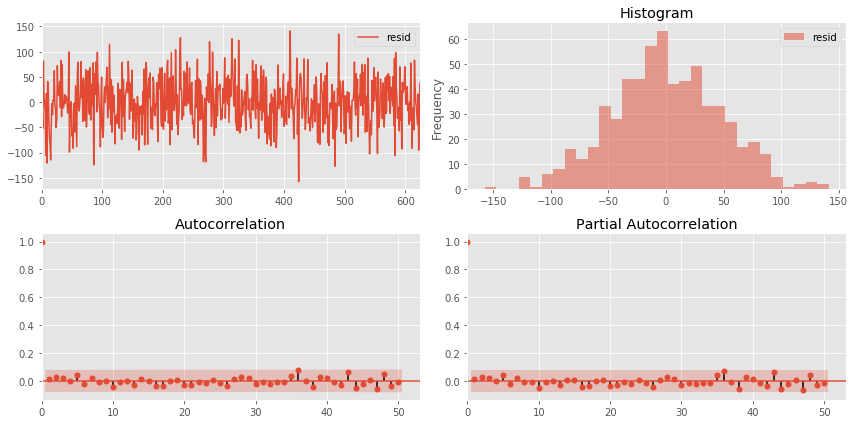The following diagram illustrates the hyperplane fitted by the model to the randomly generated data points

In :
three_dee = plt.figure(figsize=(15, 5)).gca(projection='3d')
three_dee.scatter(data.X_1, data.X_2, data.Y, c='g')
data['y-hat'] = model.predict()
to_plot = data.set_index(['X_1', 'X_2']).unstack().loc[:, 'y-hat']
three_dee.plot_surface(X_1, X_2, to_plot.values, color='black', alpha=0.2, linewidth=1, antialiased=True)
for _, row in data.iterrows():
plt.plot((row.X_1, row.X_1), (row.X_2, row.X_2), (row.Y, row['y-hat']), 'k-');
three_dee.set_xlabel('$X_1$');three_dee.set_ylabel('$X_2$');three_dee.set_zlabel('$Y, \hat{Y}$')
# plt.savefig('multiple_regression_plot.png', dpi=300);

Out:
Text(0.5, 0, '$Y, \\hat{Y}$')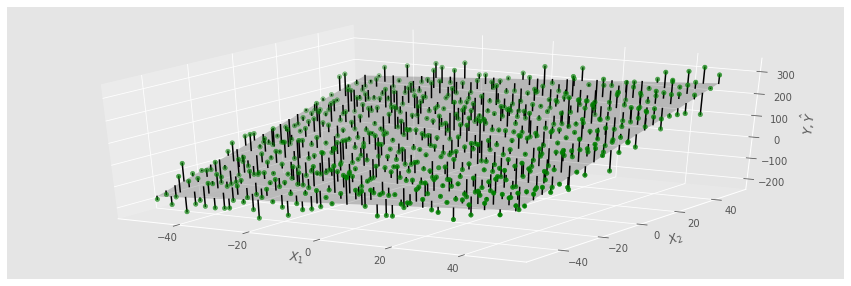#### Breusch-Pagan test for homoskedasticity¶

• High values of the test statistic (and corresponding low p-values for the test statistic) indicate the presence of heteroskedasticity.
In :
test = sms.het_breuschpagan(model.resid, model.model.exog)
print(f"Breusch-Pagan Test Statistic: {str(test)} P-Value: {str(test)}")

Breusch-Pagan Test Statistic: 0.49397711110975295 P-Value: 0.7811496333043327


For our regression, the test yields a fairly low test statistic of 0.494 (corresponding to a p-value 0.781) and thus supports our intuition that there is not heteroskedasticity present in our regression model’s residuals.

The sklearn library includes an SGDRegressor model in its linear_models module. To learn the parameters for the same model using this method, we need to first standardize the data because the gradient is sensitive to the scale.

### Prepare data¶

The gradient is sensitive to scale and so is SGDRegressor. Use the StandardScaler or scale to adjust the features.

We use StandardScaler() for this purpose that computes the mean and the standard deviation for each input variable during the fit step, and then subtracts the mean and divides by the standard deviation during the transform step that we can conveniently conduct in a single fit_transform() command:

In :
scaler = StandardScaler()
X_ = scaler.fit_transform(X)


### Configure SGDRegressor¶

Then we instantiate the SGDRegressor using the default values except for a random_state setting to facilitate replication:

In :
sgd = SGDRegressor(loss='squared_loss', fit_intercept=True,
shuffle=True, random_state=42,
learning_rate='invscaling',
eta0=0.01, power_t=0.25)


### Fit Model¶

Now we can fit the sgd model, create the in-sample predictions for both the OLS and the sgd models, and compute the root mean squared error for each:

In :
sgd.fit(X=X_, y=y)

Out:
SGDRegressor(alpha=0.0001, average=False, early_stopping=False, epsilon=0.1,
eta0=0.01, fit_intercept=True, l1_ratio=0.15,
learning_rate='invscaling', loss='squared_loss', max_iter=1000,
n_iter_no_change=5, penalty='l2', power_t=0.25, random_state=42,
shuffle=True, tol=0.001, validation_fraction=0.1, verbose=0,
warm_start=False)

As expected, both models yield the same result. We will now take on a more ambitious project using linear regression to estimate a multi-factor asset pricing model.

In :
coeffs = (sgd.coef_ * scaler.scale_) + scaler.mean_
pd.Series(coeffs, index=X.columns)

Out:
X_1     935.78
X_2   2,748.60
dtype: float64
In :
resids = pd.DataFrame({'sgd': y - sgd.predict(X_),

In :
resids.pow(2).sum().div(len(y)).pow(.5)

Out:
sgd   47.78
ols   47.78
dtype: float64
In :
resids.plot.scatter(x='sgd', y='ols');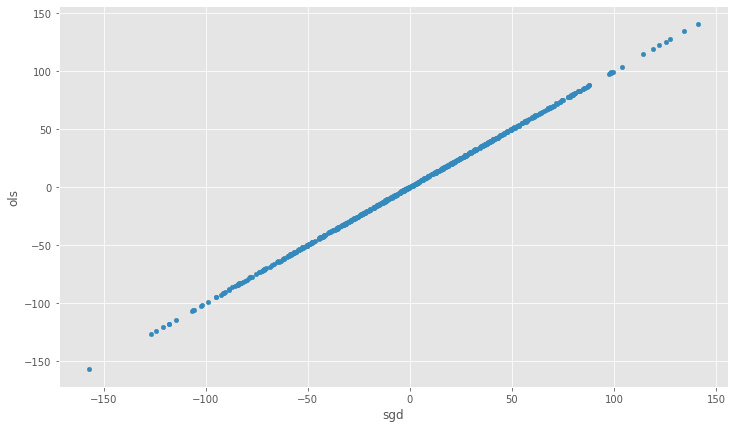In [ ]: